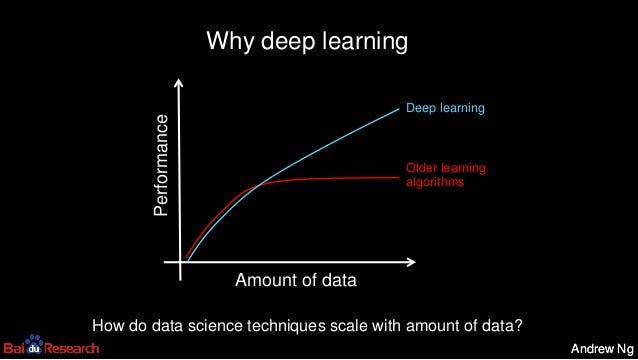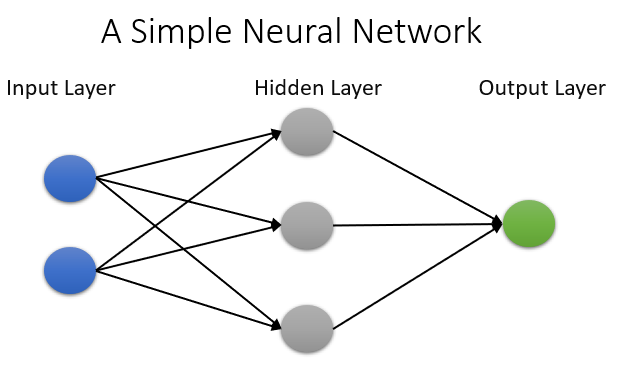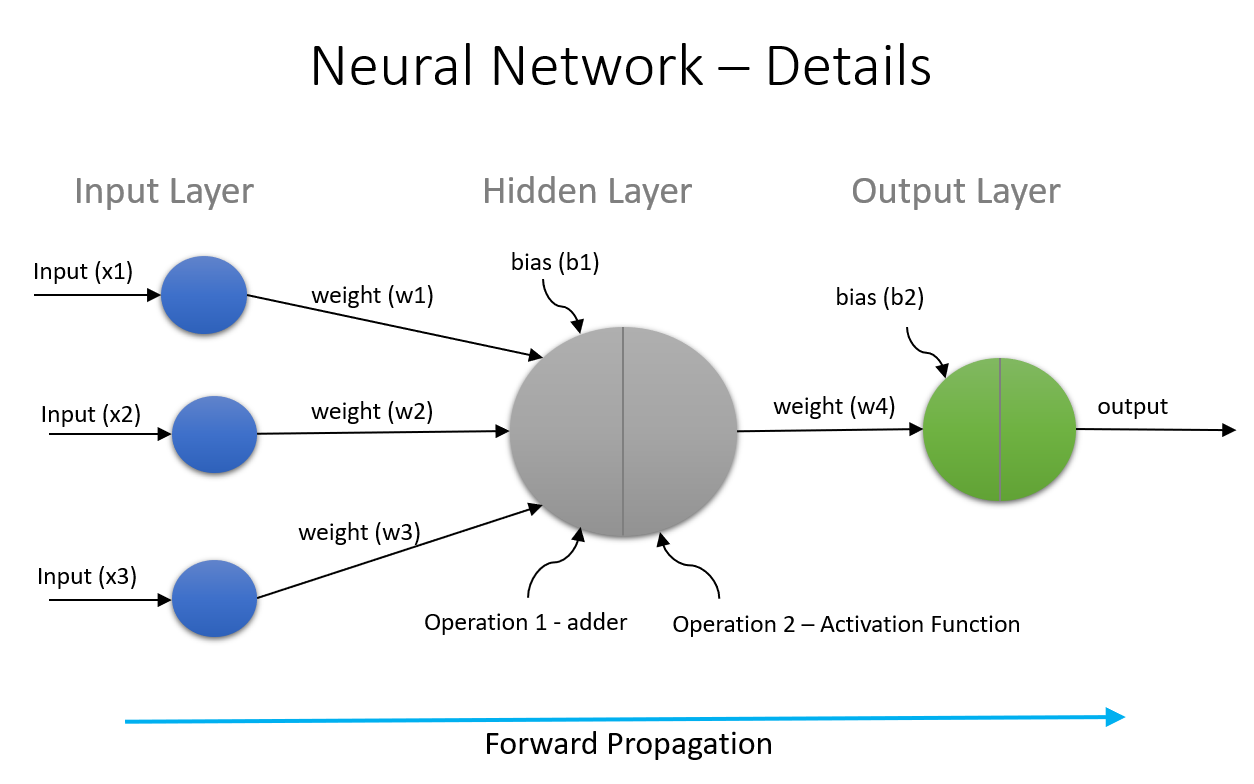# Deep Learning — A Beginners Guide

Ever wondered what is deep learning and how its changing the way we do things. In this series of tutorials, I will dig into the terminology used in the space of deep learning. A complete look at the mathematics behind activation functions, loss, functions, optimizers and much more. Along the way, I will also share links which I felt are useful rather than mentioning it to the end of the article.

Deep Learning: To put this in simple words, “Deep learning is all about making the machine to think and learn like human brains do”. In order to do this scientists came up with the concept of Neural Networks. The term “deep” refers to the depth of the network.

Andrew Ng who co-founded and led Google Brain nicely explains the concept in his 2013 talk on Deep Learning, Self-Taught Learning and Unsupervised Feature Learning. The picture below explains the need of deep learning.Figure 1 — Need of Deep Learning

Neural Networks: Try to search for neural network in google and you will end up with definition “A computer system modeled on the human brain and nervous system”. I found this definition to be more apt and simple to communicate to a layman. A neural network is a series of interconnected neurons which work together to produce the output.Figure 2 — A Simple Neural Network

The first layer is the input layer. Each node in this layer takes an input OR a feature and then passes its output to each node in the next layer. Nodes within the same layer are not connected. The last layer produces the output. The hidden layer has the neurons which have no connection to input OR output. They are activated by the inputs from nodes in previous layer.

Let us now understand some of the minute details related to the functioning of the neural network. I have put a simplified diagram below in order to explain the concepts and make the diagram less clumsy.Figure 3 — Neural Network Details

There are quite a few terms in the above diagram. Let us go through them one-by-one

Inputs (x1, x2, x3): Inputs are the input values OR features based on which the output is predicted. An example would be “Pass/Fail output (y) will be decided based on inputs Study Hours (x1), Play Hours (x2) and Sleep Hours (x3)”

Weights (w1, w2, w3): Weights indicate the strength of an input. In other words, a weight decides how much influence the input will have on the output. Considering above example, Study Hours might have higher weight compared to other two.

Bias (b1): Bias is added to neural network to take care of zero input. The bias unit ensures that neuron will be activated even in case of all zero inputs. Its important to note that this value is not influenced by previous layers. If you are aware of the linear function y = mx + c, you can relate bias to the constant ‘c’. A bias value allows to shift the activation function to the left OR right. Its explained very nicely in this stackoverflow post.

# Operations within a Neuron

The operations done by each neuron are always 2 steps.

Adder OR a Pre-activation Function: Not sure if there is definite name for it, but I am going to call the first operation as adder. In this step, summation of the products of inputs and weights is calculated. We also consider the bias during this step. Notice, that bias remains same while weights and inputs may vary. Its defined by the function below:

Considering our example above, it can be written as:

Activation Function: The activation function takes the value calculated by the adder function and turns it to a number between 0 and 1 (activated (0), deactivated(1)). The function determines whether a neuron should be activated (fired) OR not, based on neuron’s input being relevant for the model’s prediction. There are many different activation functions and I am going to cover them in follow-up posts. Below is the depiction of a Sigmoid activation function.Figure 4 — Sigmoid Activation Function

# Forward Propagation

The complete process of calculating the values in hidden layers and the output layer is called the forward propagation. This type of network is also called feed-forward network. Note that I have just showed the calculations in one hidden layer with one neuron in it. In a real neural network, there can be multiple hidden layers with multiple neurons in each layer. Same process is repeated in all layers before producing final output.

# Loss Function, Back Propagation and Optimizers

Before I introduce new concepts, its important to understand why we need them. For that, lets take an example. I am taking a single record with an output(y) of value of 1. We will build a simple neural network to predict this output based in input features x1, x2, x3.

A discussed above, the first operation is the adder function(a11) followed by the activation function(h11) in the hidden layer, which in this case translates to:

The same calculation is repeated in the output layer as below. Note that h11 is the input from hidden layer.

h21 is the output which is nothing but our Y-hat. Our true value of Y being 1.

The aim should be to bring Y and Y-hat closer so that we are accurate in predicting the output given a set of input parameters. Now its time to understand new concepts in terms of Loss Function, Back Propagation and Optimizers.

Loss Function: Simply put, Loss is the prediction error of the neural network and the method to calculate the Loss is called the Loss Function. There are many different loss functions which I will cover in follow-up topics. For now, let us use the mean squared error (MSE) loss function. Considering our example above, the MSE is:

Optimizers: Optimizers are the algorithms OR methods which are responsible for reducing the loss ( Y — Y-hat). The way to reduce the loss is by updating the weights and bias parameters. There is one more parameter called “Learning Rate” which I will introduce when I am writing about Optimizers. There are different optimizers like Gradient Descent (GD), Stochastic Gradient Descent (SGD), Adagrad, Adam etc.

# Back Propagation

The objective of back propagation is to update the weights so as to to reduce the loss (Y — Y-hat). It does this by taking into account the loss function and using optimizers to update the weights. Once we complete one set of forward propagation and back propagation, we call it an iteration. We keep repeating this until we reduce (Y — Y-hat) so that we get more accurate results. The new terminology can be visualized as below.

So here you go. This is the gist of Artificial Neural Networks (ANN). The below diagram depicting sequence of actions may help to digest things a bit.